Adapted for the Internet from:Why God Doesn't Exist
 The physical mechanism behind instantaneous action-at-a-distance
T   =         G                m  * m   / d²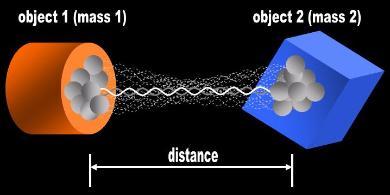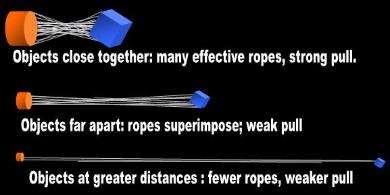Introduction

Newton's gravitational equation implies that gravity acts instantaneously across the entire Universe. The mathematicians
never figured out a physical mechanism that can simulate instantaneity. This is due, in great measure, to the fact that for
the past 400 years the birdbrains only considered particles and waves.  We need to watch a minimum of two frames in a
film to conceptualize motion. We can stare at no more than one frame in a film to conceptualize 'instantaneous.' Rather
than brainstorm new ideas, the scholars decided to scrap Newton's gravitational equation and replace it with Pastor Al's
warped space.

Here I illustrate an instantaneous mechanism that is consistent with Newton's gravitational law. The rope model of light
explains each factor in Newton's 'universal' equation and finally gives physical interpretation to Mach's Principle. Under
the rope hypothesis, Newton's equation has a limited range.

At a shorter distance from Earth, the atom will predictably have more 'effective' ropes converging upon it and at a greater
distance there will be fewer. Therefore, the weight and velocity of that particular atom is a function of distance from the
Earth, specifically a function of the inverse square of the distance. This mechanism is consistent with weight being
location-specific. In Fig. 6, I show one atom comprising part of a feather at three different distances from the Earth. The
number of effective ropes increases the closer the feather is to Earth. The ropes interconnecting the Earth and the feather
fan out at steeper angles as the feather approaches our planet. If we now roll the movie of a feather falling to Earth, the
number of effective EM ropes should increase geometrically and instantaneously in accordance with Newton's equation.
The idiots of Mathematics call this phenomenon gravitational acceleration.

Thus, we cannot rely on one-way particles and waves to illustrate concepts such as potential, weight, and velocity that
have meaning only for a particular location. These static parameters can only be simulated with a bundle of ropes
converging on a given location. The number of ropes ending in an atom is an inverse function of distance from the object
used as a reference. Gravitational potential is a phenomenon involving a certain number of invisible EM ropes binding an
atom at a given distance from the object used as a reference.

3.0   Relativists fight back

At this point, the stupid idiotic moron of Mathematics pulls rank and tells you that my argument is moot because Newton's
equation has been superseded by Einstein's field equation: Gab =kTab.  Newton was debunked over 100 years ago, so I'm
barking up the wrong tree, chasing a strawman.

So let's find out if this is really true. These stupid mommy's boys who study at Cambridge and Harvard have no street
smartness, and just parrot what their instructors drive into them.

a.       Einstein's equation carries the gravitational constant G. The value they plug for G is determined

What the idiots of Mathematics have done is borrow G from a micro scenario and extrapolated
to a macro scenario without any physical justification. General Relativity only deals with macro
scenarios (cosmic distances, warped space). If the morons are going to reject Newton and fall
back on warped space, they cannot use G because G is determined at everyday level distances.
Is warped space the reason your liver attracts your heart? In other words, GR has yet to justify
why G applies to the micro world whereas warped space doesn't.

b.       NASA does not use Einstein's equation at all for day to day operations. NASA uses classical
(i.e., Newtonian) equations to calculate orbits and escape velocities:

" Although Newton's theory has been superseded, most modern non-relativistic
gravitational calculations are still made using Newton's theory" 

[ The euphemism 'relativistic' means that an observer and/or a frame of reference
is involved. Science is objective and dispenses with witnesses and testimony
(i.e., measurement). Science has no use for observers. A frame of reference is a
surrealistic concoction of Mathematics that is outside the purview of Science.]

" The 'Flight Testing Newton’s Laws' NASA Education Series uses aircraft to
stimulate the student’s interest in the physical sciences and mathematics. The
main emphasis lies in showing how Newton’s three laws of motion apply to flight
testing an aircraft." 

" Newton gave us a mathematical description of how one object affects, and is
affected by, the gravitational force of another object. Many, many years of
observations have proven this description to be accurate (at least for masses
like those of the planets)." 

" At high altitude, where the GPS clocks orbit the Earth, it is known that the clocks
run roughly 46,000 nanoseconds (one-billionth of a second) a day faster than at
ground level... The orbiting clocks also pass through that field at a rate of three
kilometers per second--their orbital speed. For that reason, they tick 7,000
nanoseconds a day slower than stationary clocks. To offset these two effects,
the GPS engineers reset the clock rates, slowing them down before launch by
39,000 nanoseconds a day. They then proceed to tick in orbit at the same rate as
ground clocks, and the system "works." ... In (Einstein) theory, however, it was
expected that... continuously varying relativistic corrections would have to be
made to clock rates.... But these corrections were not made. Yet "the system
manages to work, even though they use no relativistic corrections after launch...
They have basically blown off Einstein" 

" Orbital mechanics or astrodynamics is the study of the motion of rockets and
other spacecraft. The motion of these objects is usually calculated from Newton's
laws of motion and Newton's law of universal gravitation, collectively known as
classical mechanics. 

Therefore, Newton is not yet quite dead as relativists would have you believe. It seems, rather,
that it is Einstein who is dead as far as routine business is concerned! The only people who
use Einstein's equations are the idiots who man the think tanks at the universities! There is no
practical use for Einstein.

c.       The idiots of Mathematics tell you that they chose Einstein's field equation over Newton's
Universal Law because it was more accurate in describing Mercury's perihelion shift.
However, Einstein's equations don't describe Mercury's perihelion exactly either.

" the predictions of general relativity perfectly account for the missing precession
(the remaining discrepancy is within observational error)." 

Van Flandern argues that Einstein fiddled with the equation to match observation:

" the choice of coefficients of potential phi in the space-time metric is arbitrary.
Einstein knew the unmodeled perihelion motion of Mercury, and therefore
confined his attention to metrics that predicted this quantity correctly." 

[If this is true, it reduces General Relativity to poppycock. The famous field equation
is ad hoc and makes Einstein's field equations even more suspicious. Perhaps we
need to take a new look at the garbage that Einstein 'invented'.]

So should we discard Einstein's equation because it does not account for Mercury's perihelion
exactly? The idiots of Mathematics were penny wise and pound foolish, that's what they were.
They abandoned substance for form. They chose a radically different and irrational physical
interpretation for gravity (warped space) that was not justified by the tiny discrepancy in the
calculations. Newton and Einstein can't both be right even at the limit (as the idiots of Math like
to say) because they have radically different physical interpretations for gravity. Therefore, if the
idiot of Math requests a full account of Mercury's perihelion discrepancy in terms of Newton's
equation, I have just as much right to request that the idiot of Math tell me why these two
equations are identical 'at the limit' if they have such widely different physical explanations for
the same phenomenon!

d.       How do you explain ocean tides with Einstein's field equations, you stupid morons? Does
warped space also flatten the Moon and the Earth? Does it squash your brains against
warped space in the process?

Here is John Baez's ridiculous explanation on behalf of the establishment:

" In the vacuum there is no energy density or pressure, so ¨ V |t=0 = 0, but the curvature
of spacetime can still distort the ball. For example, suppose you drop a small ball of
instant coffee when making coffee in the morning. The grains of coffee closer to the
earth accelerate towards it a bit more, causing the ball to start stretching in the vertical
direction. However, as the grains all accelerate towards the center of the earth, the ball
also starts being squashed in the two horizontal directions." 

[And this guy is a mathematical guru in a university somewhere in California. He is known
for his only revolutionary contribution to 'science': the Crackpot Index.]

Baez has sold you a whopper. He tells you that he's going to explain tidal waves and gives you a description: that
the grain of coffee comes closer to the Earth because it comes closer to the Earth. Great!So how does warped space
or space-time physically induce the grain to accelerate? That's what we want to know! Baez hasn't explained
anything! If he knew why a grain accelerates, we don't need the rest of his idiotic analogy! Baez is justifying the
nature of gravity by saying that gravity (i.e., warped space) 'pulls' on the grain.

Yet more ludicrous about his explanation is that it shows that he doesn't understand the fundamentals of his own
religion. Even elementary school children know the first rule of relativity:

QM deals exclusively with the micro world. GR deals exclusively with the macro world.

Baez is applying Pastor Al's idiotic warped space in a micro scenario, something which is a no no in Mathematical
Physics. Then, he has the gall to call others crackpots!

The majority of mathematicians explain ocean tides with classical equations. There is no room for Einstein there.

Again, we haven't buried Newton yet. Classical Mechanics will be dead the day the morons of relativity can explain the
simple phenomena of everyday Physics with Einstein's idiotic equations. Einstein has no authority to use big G or little c
because the former is determined in micro-scenarios and the latter cannot be a constant if it can never travel 'straight' in
Einstein's warped space. I repeat: the reason the mathematicians abandoned Newton was not because of Einstein's petty
advantage in accuracy. The reason they abandoned the old master is because they couldn't figure out a way to simulate
the instantaneous action implied by Newton's equation, especially with particles. Now we have a different proposal on the
table that does in fact model instantaneous gravitational effects, and Newton's universal equation needs to be looked at in
this new light.

4.0   Conclusions

Under the rope model of light, gravity acts instantaneously across the Universe consistent with Newton's amply tested
equation because every atom is interconnected to all others by EM ropes. Likewise, gravity permeates every physical
object because EM ropes end in atoms irrespective of their location or of the object they form a part of. You are unable to
protect yourself from gravity because the ropes from the atoms comprising the Earth are connected to you right through
the shield you hold in front of you!

" The amazing thing about gravity is that it goes through things... You're pulling on atoms
right now on the other side of the Earth" 

The rope hypothesis implies that every atom in the Andromeda Galaxy is connected to every atom in your body! If I stand
in front of you, the ropes that end in your body run right through me! The ropes do not have the ability to tangle, and this
trick of nature is the reason the mathematicians have never zeroed-in on this mechanism. Mother Nature has utterly fooled
them all!  Whenever you move your pinkie, you tug on the entire galaxy two million light years away! This phenomenon is
known as Mach's Principle (another intuition that the mathematicians discarded because they were never able to explain it
with discrete particles!). The number of 'effective' ropes impinging on the atoms of your body increases and decreases with
every step you take. The closer you are to Earth, the greater the number of 'effective' ropes converging on you from this
particular celestial object. (The question of how the ropes avoid tangling is beyond the scope of this website.)

Actually, Newton's expression cannot and does not remain constant across the Universe in the context of the rope model
of light. It is for this reason that we have phenomena such as the Pioneer anomaly and the galaxy rotation problem (both
beyond the scope of this website).

2.0   A photograph of weight

I will quickly review page 1 of this module dealing with weight as an introduction to the explanation that follows. The first
thing we must come to terms with is that Newton's gravitational equation has nothing to do with force. For the purposes
of Physics, a force is defined as a push or a pull. Force is a vector, which means that the object moves in a given direction.
This is not the case with Newton's gravitational equation. The gravitational equation depicts a static scenario. Newton's
equation has to do with tension. The distance in Newton's equation is not the distance-traveled of Mathematical Physics.
It is the distance of Physics. The distance in the numerator of the gravitational equation is a static separation between two
objects. Therefore, I will not be talking about force (F). I will be talking about a static concept most people recognize as
tension (T).

Weight is a static concept, a tension or a potential, rather than a dynamic force. Acceleration is to a movie, what weight is
to a photograph. We should be able to explain weight without invoking motion (i.e., acceleration). Newton’s gravitational
equation has to do with weight whereas his force equation (2nd Law) has to do with acceleration and velocity. The
gravitational equation does not calculate force, but rather tension (or potential). For instance, if a test object (m) is located
closer to the center of the primary mass (M), both weight and velocity of the object increase. However, weight increased
because of a change in ‘physical’ distance (the qualitative gap between the two objects decreased). Conversely, an
increase in velocity means that the object is now further from origin. Weight dispenses with both motion and time. Indeed,
we could have two identical objects simultaneously at different altitudes above the Earth with different weights. We do
not need to accelerate or move either of them in any way because, if we did, their weights would change! In Physics, we
don't measure weight. We count the number of ropes bearing down on the target! Measurement is a technique
circumscribed solely to Mathematical Physics. It has little to do with Science or with Physics because it is a subjective
technique. In Science and in Physics we conceptualize and visualize.

Gravitational potential, weight, and velocity are static concepts in the sense that these parameters have meaning only
for a particular location in space. We should be able to synthesize them in a single image. Only the rope hypothesis can
simulate conceptually static phenomena. The assumption that every atom in the Universe is connected to all other implies
that whether an H-atom is part of an elephant or part of a feather that is floating in space, it will have the same number of
ropes converging upon it from the Earth. That's what Fig. 5 depicts. You are staring at a photograph of weight (as well as
of velocity) of one atom!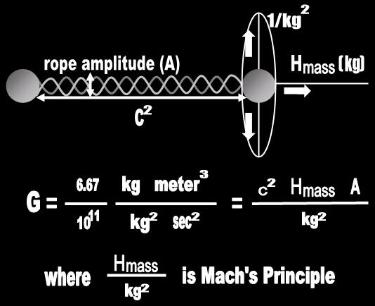(          )

We're essentially done. The worst part is over. We are not going to perform a calculation on these objects. We are not
going to multiply 'object times object,' for what sense would that make in Science?  We are attempting to do something
else. We are attempting to provide a physical explanation to each factor in Newton's equation. We are trying to visualize
the invisible mechanism of gravity in action.

So now, how do we interpret this expression in Physics? What physical significance doesmass times masshave?

Let’s look at a simple example to understand the problem the idiotic mathematical abstraction known as mass causes in
Physics. Let’s start with kindergarten arithmetic. You have two sets of three apples each. How many apples do you have?
The answer is easy. We end up with 6 apples. No problem. Everyone with kindergarten-level schooling understands this.

Now let’s complicate things a bit. Let's try to multiply the apples against each other. What does 3 apples times 3 apples
mean? How do we resolve the expression (3 apples)²? What is the physical significance of 9 apples squared? What do
the units ‘apples squared’ mean for the purposes of Physics? We cannot even imagine this under the ridiculous
corpuscular hypothesis still in use in the religion of Mathematical Physics.

Under the rope hypothesis, things quickly fall in place. The assumption is that each atom is not only connected by means
of an  EM rope to the other atoms that make up 'mass1,' but also to the atoms that make up 'mass2.'  In other words, we
will assume for simplicity that each apple is a single unit of mass and is thus bound to all other apples by exactly one
rope. Under these assumptions, the physical interpretation of (mass * mass) is that every unit of mass comprising one
object is physically bound by a rope to every unit of mass comprising the other (Fig. 2). The exponent represents the
number of ropes interconnecting the two objects. The physical significance of (9 apples squared) under these
circumstances is that every apple in Set 1 is connected to each of the three apples comprising Set 2. We have six
discrete bodies, but 9 ropes!
 Fig. 1   The (mass1 * mass2) factor
 Fig. 6   Gravitational AccelerationInstantaneous action-at-a-distance
 ension
1
2The ball bounces instantaneously consistent with my equation, Al!Actually, it doesn't, Newt. My equation indicates that the ball bounces at the finite speed of light.
 Fig. 2   mass  x  mass
 Fig. 5   WeightBrothers! Brothers! Let there be noanimosity between men of the cloth.Tension is as instantaneous as it gets. Tempers can get as tight as this rope.Perhaps there are no balls in relativity.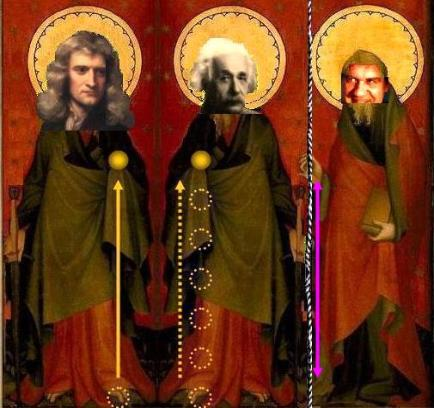Here we see two objects, eachcomprised of 4 H-atoms or apples. An H-atom is bound toeach atom in the Universe bya single rope. In this system, there are two objects, made of
 The number of 'effective'EM ropes converging onan H atom is a functionof distance from theobject.
 Here we see an atom which forms apart locations with respect to Earth.The number of 'effective' ropes converging on this atom increasesinstantaneously the closer the featheris to our planet. The effect is instantaneous because it is a functionof angles and not of traveling.
 We replace the mathematical factor (mass1 * mass2) in Newton's gravi-tational equation with two physical objects separated by distance.Now we are doing Physics and willbe able to explain scientifically why Newton's equation works. (i.e., Wewill be able to make a movie of two objects gravitationally attractingeach other instantaneously,meaning in each frame of the film.)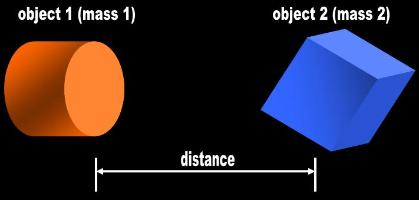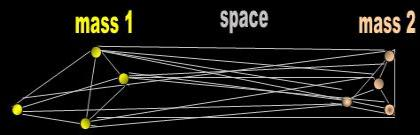4 atoms each for a total of 8 atoms, and 16 ropes interconnecting them. The 16 ropesprovide the physical significance of the expression (mass * mass) in Newton's gravita-tional equation. (The special case where there are only two lonely units of mass isbeyond the scope of this website.)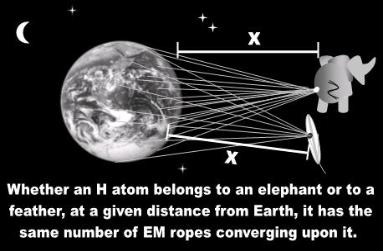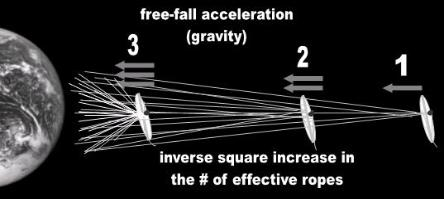Notes:1.  The reasons and logic behind some of the definitions and assumptions entertained herein     (e.g., 'effective ropes', unit of mass, how the ropes avoid tangling) are beyond the scope     of this page as are some of the claims I make. Therefore, it would be unreasonable for a     skeptic to hope to understand the entire rope model of gravity by merely reading the     material on this page. The purpose of this page is limited to providing an overview of the     theory.2.  At the bottom of the page, I have included a couple of figures to aid in the visualization of     Newton's equation. The material is copyrighted.

Perhaps in the religion of Mathematical Physics there is something called mass, but this is not the case in Science. In the
real world, there are only 3-D objects known as solids. Therefore, the first thing we need to do is convert Newtonian Math
into rational Physics if we wish to visualize what this equation stands for.

Whenever a mathematician uses the irrational word mass, the sane person supposes that he is referring to a physical body.
The juror imagines an object, something that has shape. I clarify this because it is not necessarily true in the religion of
Mathematical Physics. For example, a photon has a body, yet no mass, and a black hole singularity has mass, but no body.
The idiots further hypothesize that there are particles of mass called Higgs Bosons. The Higgs is a particle of a concept,
something like a particle of love. That's how irrational the stupid idiots of Mathematics are. Then, they tell you that their
theories have been confirmed by experiment and that all celebrities of Mathematical Physics believe in them. You reach
your own conclusions! Here I will assume that the word mass refers to a body, a shape, an object, etc.

Newton’s gravitational equation contains two 'masses' in the numerator: (mass1 * mass2). These masses are separated by
distance. Our task is simple. If 'mass' is the mathematical representation of a body, we merely have to replace the two
'masses' with two objects (Fig. 1). We visualize them as discrete entities. Therefore, they necessarily have to be contoured
by space and separated by distance.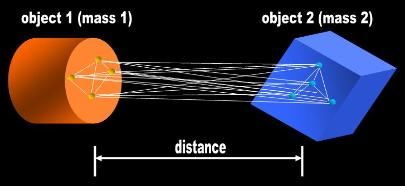Fig.  3
 We now superimpose Fig. 2 on Fig. 1.We are looking at ONLY four units of mass of each object. The objects arein reality bound by gazillions ofinvisible EM ropes that interconnectall their atoms.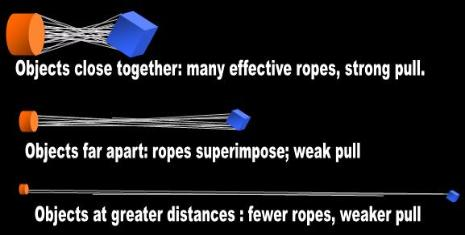Unlike one-way particles and waves, the EM rope model of light generates gravity not by traveling, but by aggregation.
The assumptions are critical: every H-atom in the Universe is connected to all others via a single, taut EM rope.

" Every atom on the Earth is pulling on every atom of you. You're also pulling on it." 

The torque signal that propagates along the rope is what we call light. The number of 'effective' ropes that arrive at an
object is therefore a function not of mathematical  distance-traveled, but of distance
(a gap between two objects).

It is now a no-brainer to visualize the effect of distance and why Newton's equation depicts gravity as acting
instantaneously across the Universe. When the two objects are very far away, all the ropes superimpose and act
essentially as one rope. When the objects are very close together, the ropes fan out and pull individually along different
angles (Fig. 4). The number of ropes interconnecting each apple doesn't change. What changes is the angle at which
they pull. At ever greater distances, more and more ropes become redundant. The changing of the angles from one
location to the next is necessarily instantaneous!
 Fig. 4Instantaneous action-at-a-distanceThe role of distance and its relation to the inverse square factor in Newton's Universal Law of Gravitation.The rope hypothesis explains(i.e., gives a physical interpretation to) why gravity follows the amply verified inverse square rule.

We can now plug the 4 units of each mass into Newton's equation:

Tension   =    G   (mass1 * mass2)  /  distance²    =    G   (4 units * 4 units)  /  distance²

However, we are not interested in numbers. We are interested in visualizing the effect of distance on the gravity between
two objects. The purpose of placing numbers in the equation was just to mathematically justify that the ropes are the
invisible agents underlying gravity and that they are also consistent with Newton's inverse square numerator. Let's now
superimpose Fig. 2 on Fig. 1 (Fig. 3).
 Instantaneous Action-at-a-DistanceClick on the tiny green atom to watch how theropes extending from the Earth reel it in________________________________________________________________________________________